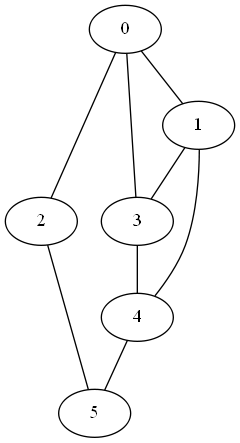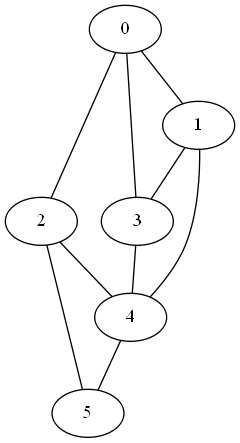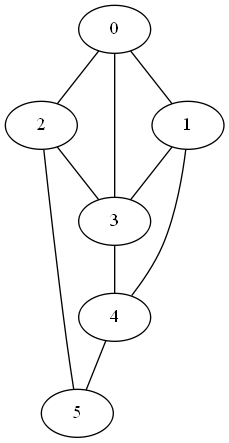# Adding an edge increases eigenvalues

When you add an edge to a graph, each of the eigenvalues of the graph Laplacian either increases or stays the same.

As I wrote in my previous post, the smallest eigenvalue is always 0. The second smallest eigenvalue is 0 if and only if the graph is disconnected. So no edge will increase the smallest eigenvalue, nor will it increase the second eigenvalue if the matrix is still disconnected after adding it. Adding some edges will have more impact on the second eigenvalue than others.The second eigenvalue of the Laplacian is 1.2679. When we connect node 2 to node 4, the second eigenvalue increases to 1.4384.If we connect node 2 to node 3 instead, the second eigenvalue goes up to 1.6972. This makes sense because node 3 is better connected than node 4. Connecting node 2 to node 3 increases the overall connectivity of the graph more than connecting it to node 4. (The second eigenvalue of the graph Laplacian is sometimes called the algebraic connectivity of the graph because it is so strongly associated with the graph’s connectivity.)Now let’s look at the rest of the eigenvalues. The full set of eigenvalues for the three graphs are given below.

```original [0.0000  1.2679  2.0000  4.0000  4.0000  4.7321]
2 -> 4   [0.0000  1.4384  3.0000  4.0000  4.0000  5.5616]
2 -> 3   [0.0000  1.6972  2.3820  4.0000  4.6180  5.3028]
```

Notice that adding an edge between nodes 2 and 4 or between nodes 2 and 3 increased some of the eigenvalues relative to their original values and left others unchanged.

Swapping the edge between nodes 2 and 4 with an edge between nodes 2 and 3 increases the 2nd and 5th eigenvalue, but lowers the 3rd and 6th.

Related post: Social networks in fact and fiction

## One thought on “Adding an edge increases eigenvalues”

1. Interesting! What’s a reference for the fact that it will affect the second eigenvalue more than others?